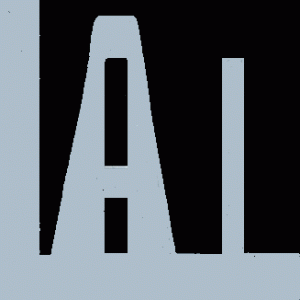# Logical Abstract Interpretation

Established: November 3, 2008

#Abstract Interpretation over Logical Formulas

Powerpoint Slides on Logical Abstract Interpretation (Lectures given in a graduate class on Static Program Analysis at UCLA and at IISc-Bangalore)

## Introduction

Logical Abstract Interpretation means performing abstract interpretation over abstract domains whose elements are logical formulas over some theory and the partial order relationship is the implication relationship (or some refinement of it). Theorem proving community has studied decision procedures for several theories. For performing abstract interpretation over logical formulas in a theory, we need more than a decision procedure. In particular, we need a join operator that can over-approximation disjunction, a postcondition operator that over-approximates existential quantifier elimination, a widen operator that guarantees convergence during the fixed-point computation process. In this project, we have shown how to build such transfer functions for a theory taking inspiration from the decision procedure for that theory. Of these, the join algorithm is typically the tricky part.

We have described logical abstract interpretation over the theory of uninterpreted functions . The decision procedure for this theory consists of performing the classic congruence closure over a data-structure called EDAG (Equivalence DAG). The join algorithm involves performing intersection of (the congurence classes of) two EDAGs. We used these transfer functions to describe the first PTIME algorithm for the classic problem of global value numbering.

We have also described logical abstract interpretation over combination of two theories (such as the combined theory of linear arithmetic and uninterpreted functions) given the logical abstract interpreter for constituent theories. The decision procedure for this theory consists of using the classic Nelson Oppen methodology for combining decision procedures. The join algorithm is more involved and requires adding some new definitions before performing join in the constituent theories.

We have also described logical abstract interpretation over universally quantified formulas over some base theory given a logical abstract interpreter for the base theory. This requires development of under-approximation algorithms for base theories.

Recently, we described logical abstract interpretation over combination of a shape/heap abstract domain with a numerical abstract domain. A heap abstract domain is more powerful than the domain of uninterested functions since it also tracks aliasing.

## Intra-procedural Analysis

• Uninterpreted Functions (SAS 2004, FSTTCS 2004)
• Combination of theories such as Linear Arithmetic and Uninterpreted Functions (PLDI 2006)
• Universally Quantified Formulas over theories such as Linear Arithmetic and Uninterpreted Functions (POPL 2008)
• Combination of a Shape Analysis with a Numerical Analysis (POPL 2009)

## Hardness Results

• Uninterpreted Functions ( SAS 2004 )
• Combination of Linear Arithmetic and Uninterpreted Functions ( ESOP 2006)
• Theories with axioms such as commutativity and/or associativity (VMCAI 2007)